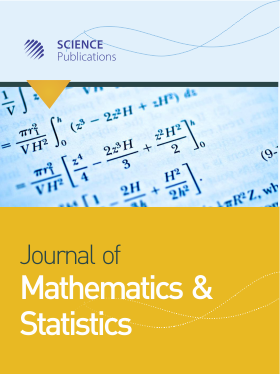Frequency: Continuous
ISSN: 1549-3644 (Print)
ISSN: 1558-6359 (Online)
Research Article Open Access

# Inverse Correlations for Multiple Time Series and Gaussian Random Fields and Measures of Their Linear Determinism

R.J. Bhansali and L. Ippoliti

## Abstract

For a discrete-time vector linear stationary process, {X(t)}, admitting forward and backward autoregressive representations, the variance matrix of an optimal linear interpolator of X(t), based on a knowledge of {X(t-j), j≠0}, is known to be given by Ri(0)-1 where Ri(0) denotes the inverse variance of the process. Let A=Is-1Ri(0)ˉ1R(0)ˉ1, where R(0) denotes the variance matrix of {X(t)} and Is an sXs, identity matrix. A measure of linear interpolability of the process, called an index of linear determinism, may be constructed from the determinant Det[Is - A], of Is - A =  Ri(0)-1 R(0)-1. An alternative measure is constructed by relating tr[Ri(0)-1]  the trace of Ri(0)-1, to tr[R(0)]. The relationship between the matrix A and the corresponding matrix, P, obtained by considering only an optimal one-step linear predictor of X(t) from a knowledge of its infinite past, {X(t-j),j>0}, is also discussed. The possible role the inverse correlation function may have for model specification of a vector ARMA model is explored. Close parallels between the problem of interpolation for a stationary univariate two-dimensional Gaussian random field and time series are examined and an index of linear determinism for the latter class of processes is also defined. An application of this index for model specification and diagnostic testing of a Gaussian Markov Random Field is investigated together with the question of its estimation from observed data. Results are illustrated by a simulation study.

Journal of Mathematics and Statistics
Volume 1 No. 4, 2005, 296-308

DOI:

Published On: 31 December 2005

How to Cite: Bhansali, R. & Ippoliti, L. (2005). Inverse Correlations for Multiple Time Series and Gaussian Random Fields and Measures of Their Linear Determinism. Journal of Mathematics and Statistics, 1(4), 296-308. https://doi.org/10.3844/jmssp.2005.296.308

• 2,104 Views